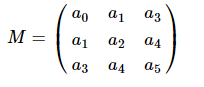# Error when using SMatrix and SVector to create symmetric matrix (TMatrixDSym)

There is a nice link with examples to create a vector and a matrix to build up symmetric matrix. However, that’s the error in my code.

For instance, I normally use these to set t he matrix elements and to see the e matrix. That part works fine.

``````TMatrixD h(3,3);
TArrayD data(9);
for (int i= 0; i < 9; i++) {
const Int_t ir = i;
const Int_t ic = 2;
data[i] = (ir*ic);
}
h.SetMatrixArray(data.GetArray());
h.Print();
``````

I also know the structure and code lines, as below, when creating the symmetric matrix from ROOT website. These lines will onnly show and set the lower diagonal of the matrix since the upper part is the same.``````unsigned int npar=3;
TMatrixDSym h2(3); //nrows=ncols  33x3 matrix
TArrayD data2(9);
for( i=0; i < npar ; ++i){
for(unsigned int j=0; j <= i ; ++j){
data2[i] =  j+i*(i+1)/2 ;
cout <<i <<"\t"  << j << "\t" << j+i*(i+1)/2 << endl ;  }}
``````

``````ERROR  when running on windowws 10. When I run my codes, I don't use  run and compile bit (.cpp++) because I get similar errrors. Normally, codes work with .x ............cpp mode.

root  .x C:\\Users\\ilker\\OneDrive\\OneDrive - harran.edu.tr\\ROOTPRO\\FindFittingValues.cpp++
Info in <TWinNTSystem::ACLiC>: creating shared library C:\\Users\\ilker\\OneDrive\\OneDrive - harran.edu.tr\\ROOTPRO\\FindFittingValues_cpp.dll
'cl.exe' is not recognized as an internal or external command,
operable program or batch file.
Error: external preprocessing failed. (0)
!!!Removing C:\\Users\\ilker\\OneDrive\\OneDrive - harran.edu.tr\\ROOTPRO\FindFittingValues_cpp_ACLiC_dict.cxx C:\\Users\\ilker\\OneDrive\\OneDrive - harran.edu.tr\\ROOTPRO\FindFittingValues_cpp_ACLiC_dict.h !!!
Basically, what’s that mean : `Limitation: Can't instantiate precompiled template ROOT::Math::SMatrix<double,3>`? What is it angry about?